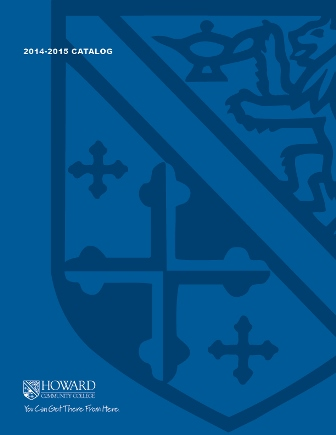2017-2018 College Catalog# MATH 070 Intermediate Algebra

The emphasis of this course is on using algebraic and graphical techniques to model and solve real world application problems. A graphing calculator is required. Topics include linear, quadratic, exponential, inverse, and logarithmic functions; rational equations (both linear and quadratic); radical and power equations; and linear and nonlinear systems.

3

### Prerequisite

An appropriate score on the mathematics placement test or a grade of A in MATH 063, a grade of B in MATH 067, a grade of C or higher in MATH 067E.

4 hours weekly

### Course Objectives

1. 1. Use problem solving strategies to explore patterns numerically, graphically, and algebraically.
2. 2. Write the equation of a linear function from a graph or a verbal problem.
3. 3. Solve rational equations.
4. 4. Model applications with linear inequalities.
5. 5. Solve absolute value equations and inequalities.
6. 6. Model applications with exponential functions.
7. 7. Solve radical equations and equations with rational exponents algebraically and graphically.
8. 8. Use the discriminant to determine the number and type of roots for a quadratic function.
9. 9. Use the concept of inverse function to explore logarithmic functions.

### Course Objectives

1. Use problem solving strategies to explore patterns numerically, graphically, and algebraically.

2. Write the equation of a linear function from a graph or a verbal problem.

3. Solve rational equations.

4. Model applications with linear inequalities.

5. Solve absolute value equations and inequalities.

6. Model applications with exponential functions.

7. Solve radical equations and equations with rational exponents algebraically and graphically.

8. Use the discriminant to determine the number and type of roots for a quadratic function.

9. Use the concept of inverse function to explore logarithmic functions.